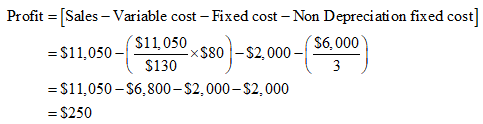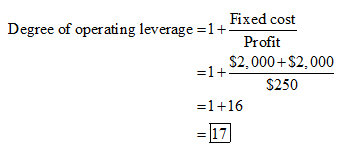# Modern Artifacts can produce keepsakes that will be sold for \$130 each. Nondepreciation fixed costs are \$2,000 per year, and variable costs are \$80 per unit. The initial investment of \$6,000 will be depreciated straight-line over its useful life of 3 years to a final value of zero, and the discount rate is 14%. a. What is the degree of operating leverage of Modern Artifacts when sales are \$11,050? (Do not round intermediate calculations. Round your answer to 2 decimal places.)b. What is the degree of operating leverage when sales are \$17,810? (Do not round intermediate calculations. Round your answer to 2 decimal places.)c. Why is operating leverage different at these two levels of sales?

Question
1 views

Modern Artifacts can produce keepsakes that will be sold for \$130 each. Nondepreciation fixed costs are \$2,000 per year, and variable costs are \$80 per unit. The initial investment of \$6,000 will be depreciated straight-line over its useful life of 3 years to a final value of zero, and the discount rate is 14%.

a. What is the degree of operating leverage of Modern Artifacts when sales are \$11,050? (Do not round intermediate calculations. Round your answer to 2 decimal places.)

b. What is the degree of operating leverage when sales are \$17,810? (Do not round intermediate calculations. Round your answer to 2 decimal places.)

c. Why is operating leverage different at these two levels of sales?

check_circle

star
star
star
star
star
1 Rating
a. Calculate the degree of operating leverage when sales are \$11,050 as follows:

Step 1: Calculate the total profit as follows:Step 2: Calculate the degree of operating leverage as follows:Therefore, the degree of operating leverage is 17.00.

...

### Want to see the full answer?

See Solution

#### Want to see this answer and more?

Solutions are written by subject experts who are available 24/7. Questions are typically answered within 1 hour.*

See Solution
*Response times may vary by subject and question.
Tagged in